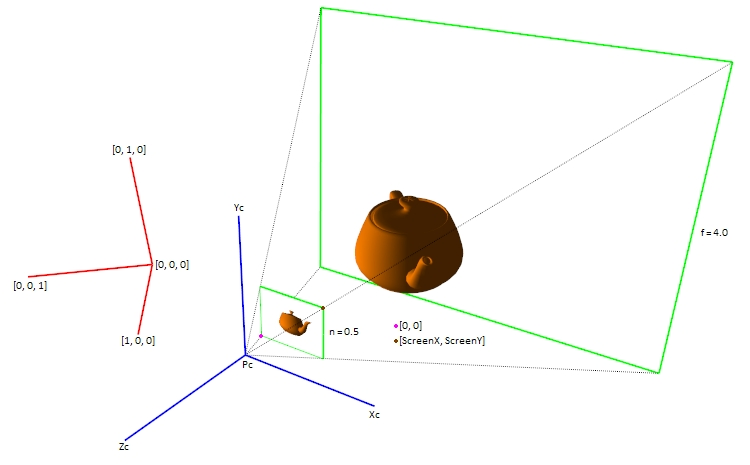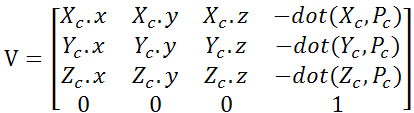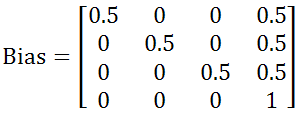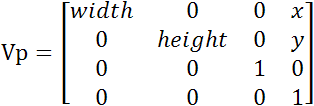3D C/C++ tutorials - Resources - OpenGL perspective projection Home Resources OpenGL Software rendering Ray tracing Downloads 3D C/C++ tutorials -> Resources -> OpenGL perspective projection Use for personal or educational purposes only. Commercial and other profit uses strictly prohibited. Exploitation of content on a website or in a publication prohibited. To compile and run these tutorials some or all of these libraries are required: FreeImage 3.16.0, GLEW 1.11.0, GLUT 3.7.6 / GLUT for Dev-C++, GLM 0.9.5.4 Getting started in Visual Studio Express 2013 mat4x4 M - model matrix - model-to-world space transformation, the result of several matrices multiplication (translation, rotation, scaling) may be stored in it mat4x4 V - view matrix - world-to-view space transformation mat4x4 P - perspective projection matrix - view-to-clip and clip-to-ndc space transformation (perspective division must be applied) mat4x4 Bias - bias matrix - ndc-to-texture space transformation (shadow mapping, screen space ambient occlusion, ...) mat4x4 Vp - viewport matrix - viewport transformation vec4 vm = vec4(x, y, z, 1) - vertex in model space vec4 vw = M * vm - vertex in world space vec4 vv = V * vw - vertex in view (camera, eye) space V causes vw to be rotated and translated in the opposite direction considering the rotation and position of the camera if vv.z < 0 then vv is in front of the camera vec4 vc = P * vv - vertex in clip space vc.x = P * vv.x vc.y = P * vv.y vc.z = P * vv.z + P * vv.w = P * vv.z + P vc.w = P * vv.z = -vv.z vc = P * V * M * vm = (P * (V * (M * vm))) - output of a vertex shader - gl_Position For performance reasons the result of the model and view matrix multiplication is stored in the modelview matrix (GL_MODELVIEW_MATRIX, gl_ModelViewMatrix) MV = V * M; and the result of the modelview and projection matrix multiplication is stored in the modelviewprojection matrix (gl_ModelViewProjectionMatrix) MVP = P * MV = P * V * M;. if vc.w > 0 then vc is in front of the camera if (-vc.w <= vc.x < vc.w) and (-vc.w <= vc.y < vc.w) and (-vc.w <= vc.z < vc.w) then vc is inside the camera frustum and is visible on the screen vc.w != 1 therefore vc must be divided by vc.w vndc = vc / vc.w - perspective division if (-1 <= vndc.x < 1) and (-1 <= vndc.y < 1) and (-1 <= vndc.z < 1) then vndc is inside the camera frustum and is visible on the screen vec4 vt = Bias * vndc - vertex in texture space vt.x = vndc.x * 0.5 + 0.5 vt.y = vndc.y * 0.5 + 0.5 vt.z = vndc.z * 0.5 + 0.5 vt.x and vt.y are used to fetch the depth texture and vt.z is used for depth comparison (shadow mapping, screen space ambient occlusion, ...) if (0 <= vt.x < 1) and (0 <= vt.y < 1) and (0 <= vt.z < 1) then vt is inside the camera frustum and if vt lies in the near clip plane then vt.z = 0 far clip plane then vt.z = 1 vt.z is used for depth test and stored in the depth buffer vec4 p = Vp * vt - pixel on the screen p.x = vt.x * width + x p.y = vt.y * height + y p.x and p.y are coordinates to the color and depth buffers if a vertex vt is inside the camera frustum then x <= p.x < x + width y <= p.y < y + height See the Simple software renderer or Software shadow mapping tutorials for more details.View matrix - gluLookAt(ex, ey, ez, cx, cy, cz, ux, uy, uz) vec3 Pc = vec3(ex, ey, ez); vec3 Zc = normalize(Pc - vec3(cx, cy, cz)); vec3 Yc = normalize(vec3(ux, uy, uz)); vec3 Xc = normalize(cross(Yc, Zc)); Yc = cross(Zc, Xc);Perspective projection matrix - gluPerspective(fovy, aspect, n, f)Bias matrixViewport matrix - glViewport(x, y, width, height)© 2010 - 2016 Bc. Michal Belanec, michalbelanec (at) centrum (dot) sk Last update June 25, 2016 OpenGL® is a registered trademark of Silicon Graphics Inc.